# Test: Nuclear & Particle Physics - 1

## 20 Questions MCQ Test GATE Physics Mock Test Series | Test: Nuclear & Particle Physics - 1

Description
Attempt Test: Nuclear & Particle Physics - 1 | 20 questions in 40 minutes | Mock test for GATE preparation | Free important questions MCQ to study GATE Physics Mock Test Series for GATE Exam | Download free PDF with solutions
QUESTION: 1

### The mass number of an element in a radioactive series is 223. The radioactive series is

Solution:

Mass number of radioactive element = 223
This mass number can be written in the form of 4n + 2, which coresponds to Uranium series.

QUESTION: 2

### A nucleus having mass number A decays by α - emission to the ground state of its daughter nucleus. The Q value of the process is E. The energy of the a - particle is

Solution:

For α -particle emission, the kinetic energy of α -particle is given by
Kinetic Energy = Q x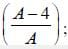where Q is energy released during α - decay process.

QUESTION: 3

### Assuming that a nucleus is a sphere of nuclear matter of radius 1.2 x A1/3 fm, the average nucleon density in S.l. units is; approximately

Solution:

The radius of nuclear matter is given by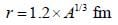The nucleon density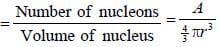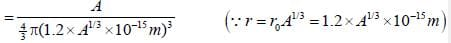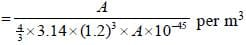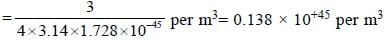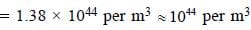*Answer can only contain numeric values
QUESTION: 4

The ratio of radii of U238 and He4 nuclei is_______________(upto one decimal place)

Solution: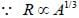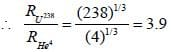*Answer can only contain numeric values
QUESTION: 5

Mass of α - particle = 4.002603 amu, mass of proton = 1.007825 amu, mass of neutron = 1.008664 amu. Taking 1 amu = 931 MeV, the binding energy of a-panicle is________(upto two decimal places).

Solution:

Binding energy o f a -particle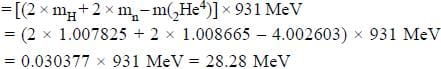QUESTION: 6

The empirical mass formula (neglecting a term representing the odd-even effect) is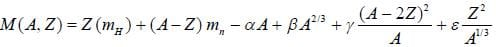where α, β, γ and ε are constants. The expression for the value of Z which corresponds to the most stable nucleus for a set of isobars of mass number A is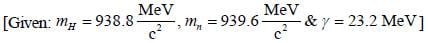Solution: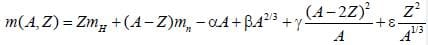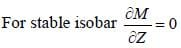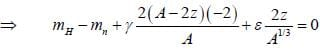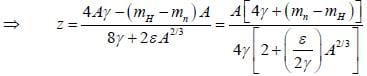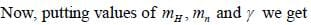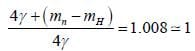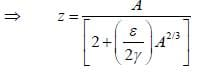QUESTION: 7

The radius of G e is measured to be twice the radius of 4Be9. The number of nucleons in Ge is

Solution: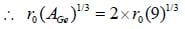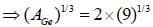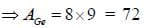QUESTION: 8

Which one of the following statement regarding neutrino is wrong?

Solution:

Neutrino has spin-1/2,. zero charge (neutral), zero rest mass and does not interact with matter.

*Answer can only contain numeric values
QUESTION: 9

The half life of radon ls 3.8 days. After 15.2 days, N/N will be________

Solution:

For Radon, T1/2 = 3.8 days
Now, number of half lives in 15.2 days = 15.2/ 3.8 = 4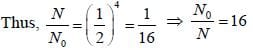*Answer can only contain numeric values
QUESTION: 10

The Q-value of the 3H(p,n)3 He reaction is -0.764 MeV. The threshold energy (for appearance of neutrons in the forward direction is___________MeV. (upto two decimal places)

Solution:

The threshold energy for apperance of neutron in the forward direction is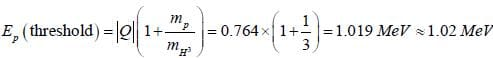QUESTION: 11

The distance of closest approach of α -particles to the Copper nucleus (Z = 29). when a-particles of 5 MeV energy are scattered back by a thin sheet of Copper, is

Solution:

Let the distance of closest approach of α - particle to copper nucleus is b.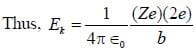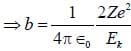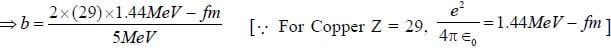QUESTION: 12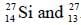Al are mirror nuclei. Tlie former is a position emitter with Emax = 3.48 MeV. The value of radius constant (r0) can be estimated as

Solution:

For positron emission,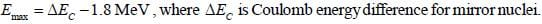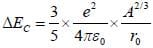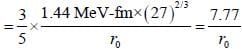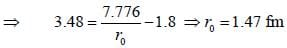QUESTION: 13

The expected value of shell-model quadmpole moment of209Bi. if the ground state spin-parity of209Bi is 9-/2

Solution:

The spin of 209 Bi 9/2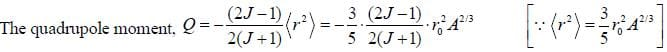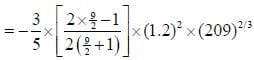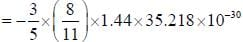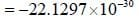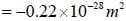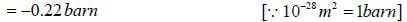*Answer can only contain numeric values
QUESTION: 14

If the binding energies of the mirror nuclei 21Sc41 and 20Ca41 are 343.143 MeV and 350.420 MeV, respectively. The radii of these nuclei by using the semi-empirical mass formula is__________fm (upto two decimal places)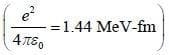Solution:

Difference in binding energies of 21Sc41 and 20Ca41 = 350.420 - 343.143 = 7.277 MeV
Since, Difference in binding energies of min or nuclei 21Se41 and 20Ca41 is equal to difference in Coulomb energies.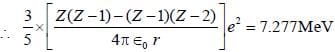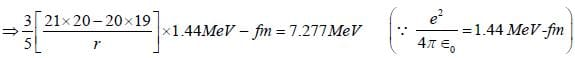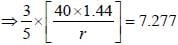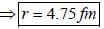QUESTION: 15

Using semi-empirical mass formula, the most stable isobar with mass number A = 65. corresponds to

Solution:

F or A = 65, the most stable isobar corresponds to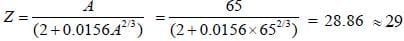QUESTION: 16

From the shell model prediction, the ground state spin and parity of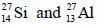nuclei, respectively.

Solution:

For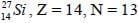The shell structure of neutrons: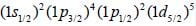Therefore, last neutrons lies in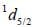shell.
Therefore, the nuclear spin of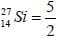The parity of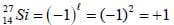Hence, nuclear spin-parity of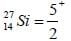Similarly for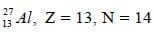The last proton lies in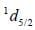shell.
Hence, the nuclear spin-parity of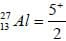QUESTION: 17

The following interactions proceed through the strong, electromagnetic (EM) or weak interactions.
(i)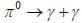(ii)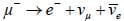(iii)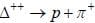The correct order of interactions is

Solution: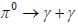is allowed via EM interaction because photon is involved.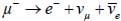is allowed via weak interaction because neutrino (Lepton) is involved.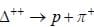is allowed via strong interaction because Barvons are involved.

QUESTION: 18

Which one of the following reaction is NOT forbidden ?

Solution:

(a) forbidden because energy is not conserved.
(b) forbidden because Baryon number is violated.
(c) allowed by strong interaction because ΔS = 0 and other quantum numbers are conserved.
(d) forbidden because Lepton number is not conserved.

QUESTION: 19

Consider the decay of K° meson of momentum P0 into ,π+ and π- of momenta p+ and p_ m the opposite direction such that p+ = 2p_. The value of p0 is given by

Solution: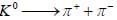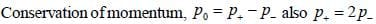∴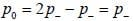i.e.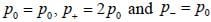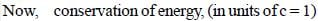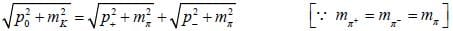Squaring both sides,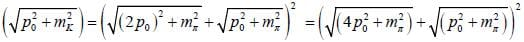⇒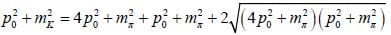⇒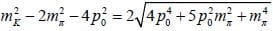Squaring again,
⇒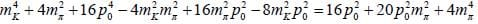⇒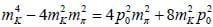⇒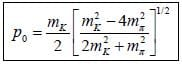*Answer can only contain numeric values
QUESTION: 20

The ground state magnetic moment of 33S16 is_____________μN.

Solution:

The nucleus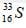has 16 protons and 17 neutrons.
The shell structure of neutrons: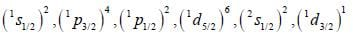Since, last neutron lies in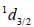shell.
Therefore, the nuclear spin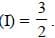The magnetic moment of last neutron in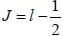state is given by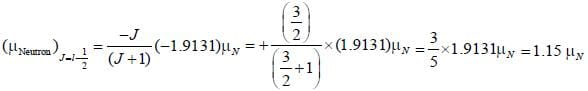Use Code STAYHOME200 and get INR 200 additional OFF Use Coupon Code